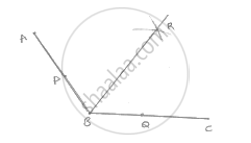# Using Your Protractor, Draw an Angle of Measure 108°. with this Angle as Given, Draw an Angle of 54°. - Mathematics

Using your protractor, draw an angle of measure 108°. With this angle as given, draw an
angle of 54°.

#### SolutionSteps of construction:
1. Draw an angle ABC of 108°
2. With center B and any radius, draw an arc which intersects AB at P and BC at Q
3. With center P and Q and radius more than 1/2PQ draw two arcs, which intersect each other at R.
4. Join BR
∴∠RBC = 54°

Concept: Basic Constructions
Is there an error in this question or solution?

#### APPEARS IN

RD Sharma Mathematics for Class 9
Chapter 16 Constructions
Exercise 16.2 | Q 3 | Page 9

Share